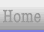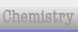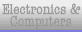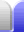# Fibonacci Sequence and the Golden Ratio

### Fibonacci Sequence

This is a sequence of numbers named after Leonardo of Pisa, who was known as Fibonacci.

0, 1, 1, 2, 3, 5, 8, 13, 21, 34, 55, 89, ...

x0 = 0
x
1 = 1
x
n = xn-1 + xn-2

Interestingly, the sum of any ten consecutive Fibonacci numbers is always divisible by 11. The number of elevens in these sums are themselves the Fibonacci numbers from 8 upward.

0 + 1 + 1 + 2 + 3 + 5 + 8 + 13 + 21 + 34 = 88 = 11 x 8

1 + 1 + 2 + 3 + 5 + 8 + 13 + 21 + 34 + 55 = 143 = 11 x 13

1 + 2 + 3 + 5 + 8 + 13 + 21 + 34 + 55 + 89 = 231 = 11 x 21

### Golden Ratio

The higher the Fibonacci numbers, the closer the ratio between two consecutive numbers is to the golden ratio, φ (the Greek letter phi). In other words, 34 / 21 is closer to φ than 21 / 13 is. (This actually happens with any similarly additive sequence except one starting with 0, 0.) The ratio of Fibonacci numbers F25001 and F25000, each of which is over 5,000 digits long, gives a value of φ accurate to over 10,000 decimal places.

However, φ is actually an irrational number – a number which can't be exactly defined by an ordinary fraction (ie, by dividing one number by another – which means it never ends, like π or √2. The value of φ can, however, be found using the square root of 5:

φ = ( 1 + √5 ) / 2

φ is called the golden ratio because it conforms to the following rules: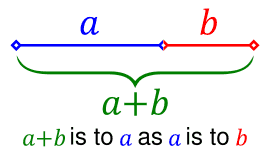φ = ( a + b ) / a = a / b 1 / φ = φ - 1 1 - φ = -1 / φ φ2 = φ + 1 (or more generally) φn+1 = φn + φn-1

φ is about 1.6180339 and 1/φ is thus about 0.6180339. (BTW, this order of accuracy is reached within the first 20 Fibonacci numbers.)

### Examples

 Strangely enough, the golden ratio also appears in a regular (meaning "even") five pointed star (known as a pentagram). The coloured line segments are in golden ratio lengths relative to each other. Each intersection of edges breaks other edges in proportions of the golden ratio.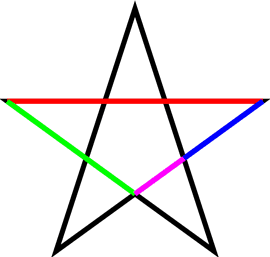A Fibonacci spiral is made from using the Fibonacci numbers to make quarter-circle curves with radii defined by the Fibonacci numbers, and linked in a spiral. The spiral at the right uses the Fibonacci numbers 1, 1, 2, 3, 5, 8, 13, 21, 34.The Fibonacci spiral is a good approximation to an ideal logarithmic spiral based on the golden ratio. (Note that not all logarithmic spirals are related to the golden ratio.) In the diagram, the Fibonacci spiral is green and the logarithmic spiral is red. Where they overlap is yellow. They are obviously very close, and the Fibonacci spiral is much easier to draw than a true logarithmic spiral.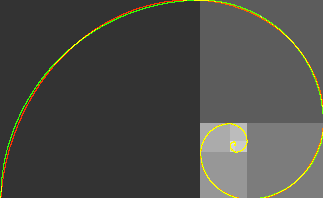Home Astronomy Chemistry Electronics & Computers Mathematics Physics Field Trips Turn on javascript for email link